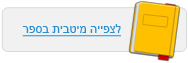עמוד:15 The marble’s potential energy is not converted into kinetic energy all at once , but rather gradually . Look at the illustration and perform the exercise written below it . Describe the gradual transformation of the marble’s energy while it rolls down the slope . Experiment B : Pendulum in motion Objective : To observe and analyze the energy transformations in a swinging pendulum . Equipment : A simple pendulum with a weight attached to its end . Procedure : Take a simple pendulum with a weight at its end . Raise the weight , and then release it . Watch it until it reaches its maximum height at the opposite side . Describe the transformation of energy that occurred from the moment you released the weight until it reached its maximum height on the opposite side . Results : Describe the changes in the pendulum’s movement . Explanation : Here too , the transformation of energy may be described using the energy diagram . This time the transformation is a bit more complicated . The description of the energy transformation is as follows : The weight’s potential energy Transforms into the weight’s kinetic energy Transforms into the weight’s potential energy Questions Is there a connection between the initial height of the pendulum and the time that elapses until it comes to a stop ? 1 . Formulate a hypothesis about the connection between the initial height of the pendulum and the time that elapses until it comes to a final stop . 2 . Plan an experiment to verify the hypothesis , using the equipment at your disposal . 3 . Perform the experiment you planned . 4 . Repeat the experiment several times ( at least twice more ) . Explain why it is necessary to repeat the experiment several times . 5 . Describe your results . 6 . Formulate a conclusion in light of the results you obtained .© מטח - המרכז לטכנולוגיה חינוכית